# RS Aggarwal Class 10 Solutions Chapter 16 Co-ordinate Geometry Ex 16D

## RS Aggarwal Class 10 Solutions Chapter 16 Co-ordinate Geometry Ex 16D

These Solutions are part of RS Aggarwal Solutions Class 10. Here we have given RS Aggarwal Solutions Class 10 Chapter 16 Co-ordinate Geometry Ex 16D.

Other Exercises

Question 1.
Solution:
Points are A (-1, y), B (5, 7) and centre O (2, -3y).
Points A and B lie on the circle with centre O.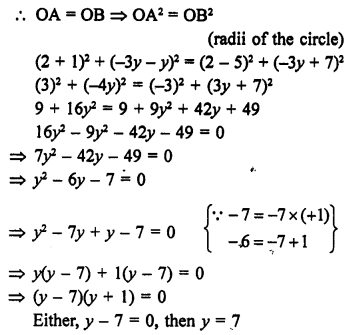or y + 1 = 0, then y = -1
y = -1, 7

Question 2.
Solution:
Point A (0, 2) is equidistant from B (3, p) and also from C (p, 5)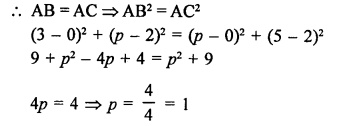Question 3.
Solution:
Three vertices of a rectangle ABCD are B (4, 0), C (4, 3) and D (0, 3)
Its diagonal are equal.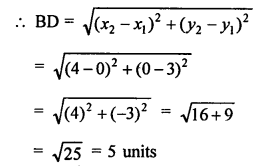Question 4.
Solution:
Point P (k – 1, 2) is equidistant from two points A (3, k) and B (k, 5)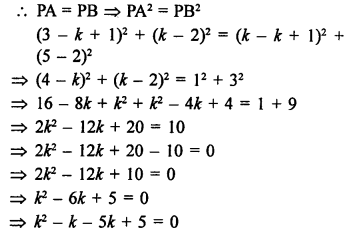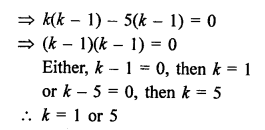Question 5.
Solution:
Let P (x, 2) divides the join of A (12, 5) and B (4, -3) in the ration m : n.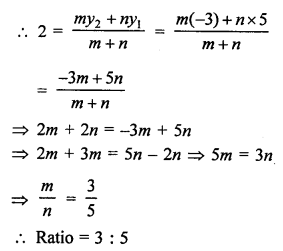Question 6.
Solution: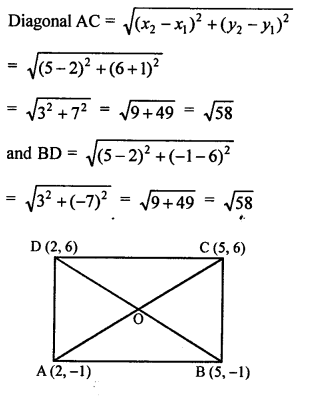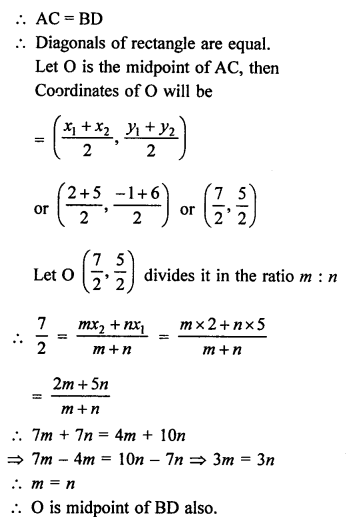Question 7.
Solution:
Vertices of ∆ABC are A (7, -3), B (5, 3) and C (3, -1)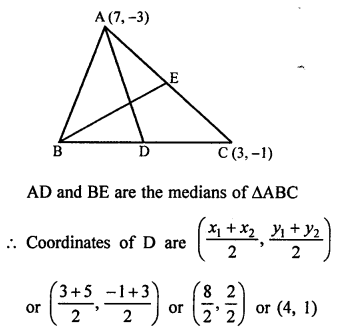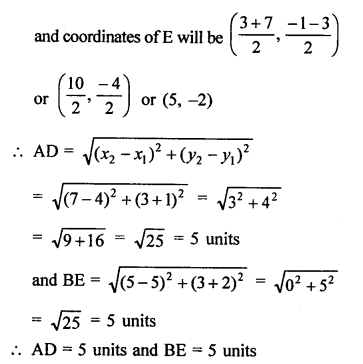Question 8.
Solution:
Point C (k, 4) divides the join of A (2, 6) and B (5, 1) in the ratio 2 : 3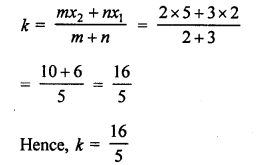Question 9.
Solution:
Let a point P (x, 0) on x-axis is equidistant from two points A (-1, 0) and B (5, 0)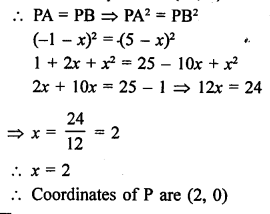Question 10.
Solution:
Distance between two points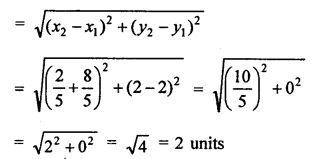Question 11.
Solution:
The points (3, a) lies on the line 2x – 3y = 5
It will satisfy it.
2 x 3 – 3 x a = 5
6 – 3a = 5 => 3a = 6 – 5 = 1
a = $$\frac { 1 }{ 3 }$$

Question 12.
Solution:
Points A (4, 3) and B (x, 5) lie on the circle with centre O (2, 3)
OA = OB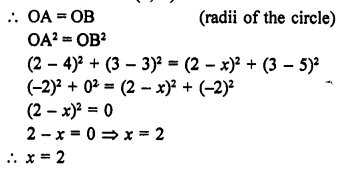Question 13.
Solution:
P (x, y) is equidistant from the point A (7, 1) and B (3, 5)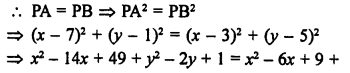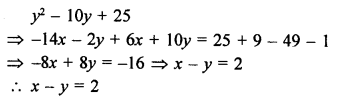Question 14.
Solution:
O (0, 0) is the centroid of ∆ABC whose vertices are A (a, b), B (b, c) and C (c, a)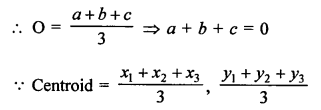Question 15.
Solution:
Coordinates of centroid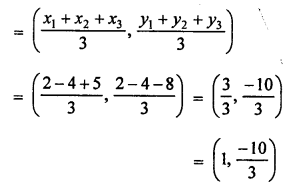Question 16.
Solution:
Let point P (4, 5) divides the join of A (2, 3) and B (7, 8) in the ratio m : n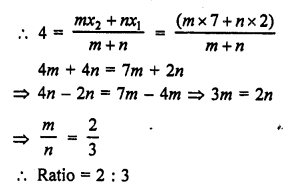Question 17.
Solution:
Points are given A (2, 3), B (4, k) and C (6, -3)
Points are collinear.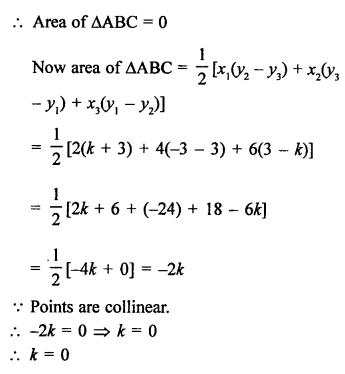Hope given RS Aggarwal Solutions Class 10 Chapter 16 Co-ordinate Geometry Ex 16D are helpful to complete your math homework.

If you have any doubts, please comment below. Learn Insta try to provide online math tutoring for you.